Reach Us+441474556909
Parametric Electrical Modelling of Human Forearm Simulation Response Using Multi-Frequency Electrical Bioimpedance | OMICS International
Journal of Biosensors & Bioelectronics

Like us on:

All submissions of the EM system will be redirected to Online Manuscript Submission System. Authors are requested to submit articles directly to Online Manuscript Submission System of respective journal.

# Parametric Electrical Modelling of Human Forearm Simulation Response Using Multi-Frequency Electrical Bioimpedance

Anand G*, Lowe A and Al-Jumaily AM

Institute of Biomedical Technologies, Auckland University of Technology (AUT), Auckland, New Zealand

*Corresponding Author:
Gautam Anand
Institute of Biomedical Technologies, AUT University
Auckland, New Zealand
Tel: 642108406290
E-mail:[email protected]

Received April 18, 2016; Accepted May 16, 2016; Published May 26, 2016

Citation: Anand G, Lowe A, Al-Jumaily AM (2016) Parametric Electrical Modelling of Human Forearm Simulation Response Using Multi-Frequency Electrical Bioimpedance. J Biosens Bioelectron 7: 206. doi:10.4172/2155-6210.1000206

Copyright: © 2016 Anand G, et al. This is an open-access article distributed under the terms of the Creative Commons Attribution License, which permits unrestricted use, distribution, and reproduction in any medium, provided the original author and source are credited.

Visit for more related articles at Journal of Biosensors & Bioelectronics

#### Abstract

This work presents a parametric electrical modelling of the electrical response of human forearm tissues through a simulation of Multi-frequency Electrical Bioimpedance analysis (MF-EBIA). The objective is to estimate an electrical equivalent representation for tissue response in terms of resistance and capacitance values for three tissue layers in the forearm – the fat, muscle and artery, using parametric fitting analysis. Following up from a simulation study of the human forearm model using Ansys® High Frequency Structure Simulator (HFSS), this work assumes an electrical model of the human forearm section for every tissue and calculates the electrical parameters. The tissue model was considered to be isotropic with regards to the dielectric properties and the consideration of blood flow was realised by taking three instances of radial artery diameter. The proposed model was validated by using the obtained values of model components to reproduce the overall response. The obtained values of resistance and capacitance for every tissue domain provide an insight into their significant contribution to the overall electrical response, which can be important while analysing their individual electrical behaviour and also helpful in various pre-experimental studies related to dielectric characterization of living tissues.

#### Keywords

Bioimpedance; Human forearm; Electrical modelling; Dielectric properties

#### Introduction

The electrical property of tissues has been the subject of research for several decades. It is generally characterized by bioimmittance (impedance and admittance) or more commonly, bioimpedance. The basis of bioelectrical response of tissues is realized through complex immittance defining the frequency dependence of their dielectric properties. The complex immittance can further be divided into complex conductivity (σ*) and complex permittivity (ε*) and their dependence on frequency explains most of the dielectric behaviour at tissue and cellular levels . The theory of tissue impedance modelling finds roots in different postulations such as Debye relaxation, Maxwell- Wagner effects and Cole theory. Debye relaxation is an important theory for identifying the dielectric, frequency dependent properties of materials based on the time constant of the model . Maxwell-Wagner effects explain the heterogeneity of biomaterials such as tissues through analysing the dielectric behaviour at the tissue interfaces . The Cole theory forms the basis of multi-frequency bioimpedance measurements and is widely acknowledged in the form of Cole-Cole systems and Cole-Cole plots . Cole and Cole provided a useful means to represent the tissue dielectric behaviour variation with frequency through the following equation :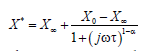(1)

where X represents conductivity (σ) or permittivity (ε) or resistivity (ρ) at zero or infinite frequency (denoted by the subscript), X* is the complex conductivity or permittivity or resistivity, ω is the frequency, τ is the mean relaxation time of the system and α is the relaxation coefficient. The value of α defines the distribution of system relaxation times. The system with α=0 behaves as a Debye response system. The general Cole equation for bioimpedance analysis can be written in terms of impedance (Z) and resistance values (R) as: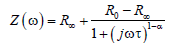(2)

where Z(ω) is the impedance, R∞ is the resistance at infinity (which is thought to reflect the resistance of extra-cellular water for bioimpedance analysis at cellular domain) and R0 is the d.c. resistance (reflecting the resistance of extra and intra-cellular water). The above equation can be represented through a Wessel diagram as a plot of resistance (R) vs reactance (X) varying with frequency and is known as a Cole-Cole plot (Figure 1).

The variation occurs as a semi-circular plot with increasing frequency. The points R∞ and R0 are the intersection points of the curve at real axis. The center of the plot lies on or below the real axis (depending on the value of α). The maximum reactance for the system is obtained at a frequency known as the ‘Characteristic Frequency’ of the system (ωc).

Since different biological systems can have different types of distribution of relaxation times, the amount of dispersion can be estimated by examining the dielectricity of the material with varying frequency. Schwan was the first to study the electrical parameters of tissues in terms of conductivity and permittivity and their frequency dependence . The behaviour was divided among three dispersion regions – α dispersion (10 Hz – 10 kHz), β dispersion (10 kHz – 100 MHz) and γ dispersion (100 MHz – 100 GHz) regions. The frequency region within 1 kHz and 10 MHz is particularly important since it is reflective of most of the passive electrical behaviour due to tissue conductivities and cellular membranes occurring mainly due to β dispersion (Figure 2).

In this research, a basic electrical model defining the response of three layers of tissues – fat, muscle and blood has been considered. A Debye-type model has been used to parametrically fit simulated bioimpedance responses. There is not an extensive literature on the electrical modelling of tissues, mainly due to the difficulty in mimicking tissue behaviour in all the dispersion regions. The use of simple circuits may be used to describe a tissue response at a single frequency . Such cases may be useful in applications of impedance cardiography or body water/fat estimation which employ a single frequency measurement analysis. However, the individual dielectric behaviour of each tissue is different, owing to different complex conductivities and permittivities and hence such a model is not likely to behave well over a wider range of the β dispersion region. It is not simple to construct an equivalent electrical circuit for three tissue layered response over a significant range of frequencies. This work presents a parametric fitting model for simulated bioimpedance measurements and a comparison of the model estimated fit with the bioimpedance measured from a numerical simulation.

#### Model

This study comprised of two levels of modelling. The first model constituted an equivalent circuit to describe the electrical behaviour of the tissues under investigation. The second level was a numerical simulation model to define the equations relating different parameters and parametrically fitting the results to reproduce the original simulation data. The construction of the equivalent circuit considered here to mimic the tissue response has been based on Debye theory. The three layers of the tissues were lumped into two domains – artery (blood) and combined fat and muscle. Each domain was individually defined through its equivalent circuit consisting of two resistors and a capacitor as shown in Figures 3a and 3b.

The circuit elements have been considered to be frequency independent, hence implicating a Debye case. The overall impedance of the two domains can be defined as: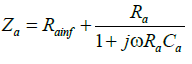(3)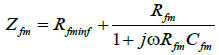(4)

where Za and Zfm are the impedances of artery and fat/muscle domains respectively, Rainf and Rfminf represent the artery and fat/ muscle resistances at infinitely high frequency, Ra and Rfm represent the resistances of artery and fat/muscle at zero frequency, Ca and Cfm represent the capacitances of artery and fat/muscle domains respectively and ω = 2πf, with f as the frequency of operation. The division of tissue layers into two domains was decided after analysing the current conduction pathways through all the three layers. The artery (blood) possesses a higher conductivity and lower relative permittivity thereby allowing for most of the current to flow through. Each of the fat and muscle layers account for variations in their conduction due to ambiguity in the current entry and exit through the layers. The artery has been modelled as passing through both muscle and fat regions, which makes it difficult to separate the conduction pathways for fat and muscle layers. Hence the fat and muscle layers have been defined here as a single domain with an impedance Zfm.

This complexity of conduction pathways was established through a simulation study of impedance measurement on a human forearm section using Ansys® HFSS (High Frequency Structure Simulator) . The constructed model was composed of fat, muscle and artery (blood) layers with frequency dependent dielectric properties [9-12]. The simulation results were obtained over a frequency range of 1 kHz to 2 MHz with three different arterial diameters – 2.3 mm, 2.35 mm and 2.4 mm to represent blood flow. Bioimpedance was simulated as being measured through a tetrapolar electrode configuration, with the external electrodes supplying current [13,14]. Bioimpedance results were plotted as Cole plots (resistance (R) vs reactance (X)) with increasing frequency. The electric field distribution and simulated bioimpedance can be observed in Figures 4 and 5 respectively.

Figure 4: Electric Field distribution in (a) Transverse and (b) Longitudinal views for the simulation model at 50 kHz.

These results were used as data sets to compute the equivalent circuit model parameters. The investigation of the electric field distribution, which also relates to the path of current distribution, revealed that the current initially entered through fat/muscle domain and divided into the two domains as it traversed its path back to the source (Figure 4).

This led to the formulation of overall tissue impedance (Ztissue) as: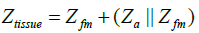(5)

The Cole plot can lead to estimating different parameters which can characterize the dielectric response of most biomaterials. The extreme values of the plot (R0 and R) along with the characteristic frequency (ωc) offer enough information to reproduce the complete behaviour of the system. Therefore, these parameters were estimated and used to calculate the model parameters defined by the passive components. The real and the imaginary values of tissue impedance were related in terms of model parameters and frequency. The obtained expressions reduce to more simplistic forms by deriving different equations for real and imaginary parts at zero and infinite frequencies such as: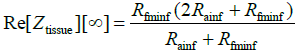(6)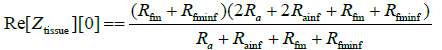(7)

The second level of modelling included numerical simulations and the parameter estimation was achieved by considering the equations (6) and (7) for three different diameters. It was assumed that no significant changes occur in the fat/muscle domain due to the diameter changes in artery, mainly due to negligible change in the artery dimensions as compared to the entire model. Hence a single value was estimated for fat/muscle domain (for both zero and infinite frequencies) as Rfm and Rfminf whereas the arterial resistances were obtained for three diameters as Ra[d1], Ra[d2], Ra[d3], Rainf[d1], Rainf[d2] and Rainf[d3], where d1 = 2.3 mm, d2 = 2.35 mm and d3 = 2.4 mm.

The simulation data was fitted as Cole equations with the centre assumed to be at real axis for all the diameters over the measured frequency range. The equations relating the reactance (X) to the resistance (R) were calculated to be: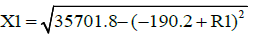(8a)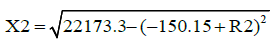(8b)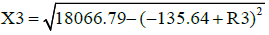(8c)

where, X1, X2 and X3 are the reactances for diameters d1, d2 and d3 respectively and R1, R2 and R3 are their respective resistances. The equations 8a, 8b and 8c were used to calculate the points of interest that included R0 [d1, d2 and d3], R [d1, d2 and d3] (the extreme points of the Cole plot) and the characteristic frequency. The parameters for the above equations were chosen to optimize their representation of the simulation data by performing a polynomial fit (Figure 6).

In order to relate the different resistance values accounting for different diameters, a term called resistance ratio was introduced. It was hypothesized that the variation in the resistance at different diameters (especially for arterial volume changes) was a function of their diameter ratio. As is well established, an increase in the cross-section decreases the resistance value (for constant length) and hence the function constitutes an inverse relation of the diameters. Hence, the resistance ratio between diameters d1 and d2 was defined as: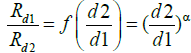(9)

where α is the coefficient describing the relation between change in electrical resistance and change in diameter values. The consideration of the above relation led to reducing complex multiple equations for different diameter parameters. The values of Ra [d1], Ra [d2] and Ra [d3] were hence computed following the above relation.

#### Results and Discussion

The above model equations were used to estimate the equivalent circuit model passive components and their relation in terms of frequency. Table 1 lists the estimated extreme value points and the characteristic frequencies for all three diameters circuit model passive components and their relation in terms of frequency. Table 1 lists the estimated extreme value points and the characteristic frequencies for all three diameters Table 1.

R0 (Ω) R∞ (Ω) Fc (kHz)
Diameter – d1 379.198 1.3 690.75
Diameter – d2 299.064 1.255 672.9
Diameter – d3 270.056 1.23 693.6

Table 1: Estimated R0, R and Fc values for three diameters.

The values of all the unknown variables were calculated using the before mentioned relations. Following an inverse relationship with the diameter changes, the overall resistances as well as the individual resistance values for different domains were calculated to be decreasing with increasing diameter. The approximation of the values of α was done to lie within 50-100 and proper relations were obtained at α = 72. The approach was heuristic due to scarcity of knowledge relating electrical response as a result of structural and mechanical changes in human arteries. The overall estimation of the equation variables was followed with the estimation of the frequency response for all the three diameters. Figure 7 shows the plots of the calculated data along with the original simulation measurements (Figure 7).

Figure 7: Overall model estimated tissue response in comparison with simulation response for three diameters.

The efficacy of the computation was analysed by adopting a Bland Altman analysis. The limits of agreement were chosen to be within 95% Confidence Intervals (CI) (±1.96*SD (Standard deviation)). Figures 8a- 8c show the correlation and Bland Altman plots for the three diameters. A promising agreement was observed between the simulation and model derived data for all the three diameters with r = 0.9993, 0.9953 and 0.9854 for d1, d2 and d3 respectively (p < 0.001 in all cases). The correlation plot also lists the linear regression equation along with sum of squared errors (SSE) for each case Figures 8a-8c.

Figure 8a: Correlation and Bland Altman plots for comparing model and simulation data for diameter d1.

Figure 8b: Correlation and Bland Altman plots for comparing model and simulation data for diameter d2.

Figure 8c: Correlation and Bland Altman plots for comparing model and simulation data for diameter d3

On the basis of above results, the present model was been found to reproduce the original data within agreeable limits. However, the performance of the system depends on the fit parameters. Quality of fit was found to be particularly sensitive to resistance ratio and consequently on the value of α. Further work needs to be done to investigate the relationship between this parameter and the physical system.

The values of Ra [d1], Ra [d2] and Ra [d3] were found to follow a decreasing trend with increasing diameters, which is expected. The consideration of Rfm to be constant for all the diameter changes appears reasonable in that it preserves the ability of the equivalent circuit model to predict behaviour. However, a more distributed model study could significantly change the metrics. The consideration of each tissue component as layered allows to represent each domain using passive components, whereas, tissue perfusion might be difficult to realise in terms of individual tissue behaviour. The equivalent circuit model also does not capture the very low-frequency response (at nearly constant reactance) apparent in Figure 5. This is likely to be due to the non-linear frequency dependence of the tissues, as modelled in the numerical simulation, which cannot be adequately represented by linear circuit elements.

This modelling presents an initiative towards quantifying the dielectricity of human limbs and estimating appropriate electrical equivalents for further analysis. There are various other factors like tissue anisotropy and inhomogeneity that play a vital role in accurately defining the overall response which have not been considered in this work. One of the works presented an electrical analogy of the inertial effects of blood flow and its non-Newtonian behaviour in human arterial system . However, the work only limited itself to the blood oscillatory flow in the arteries by constructing an electrical analog based on an electrical delay line representing the arterial hierarchy. The modelling in the present work represents a multi-frequency representation of the human forearm simulation response using passive components.

#### Conclusion

The electrical response of the human forearm (artery, muscle and fat tissue layers) has been modelled through estimating parameters of an equivalent circuit to simulation responses. The tissue layers were considered as two domains and were modelled with the consideration of three arterial diameters to mimic blood flow. The values of the model passive components were computed by estimating Cole parameters from simulation data. Good agreement was obtained between the simulation data and the model estimated response.

#### Acknowledgement

The authors would like to acknowledge the financial support provided by the Institute of Biomedical Technologies (IBTec), AUT University.

#### References

Select your language of interest to view the total content in your interested language

### Article Usage

• Total views: 9226
• [From(publication date):
June-2016 - May 26, 2019]
• Breakdown by view type
• HTML page views : 9051
• PDF downloads : 175

## Post your commentCan't read the image? click here to refresh
###### Peer Reviewed Journals

Make the best use of Scientific Research and information from our 700 + peer reviewed, Open Access Journals

International Conferences 2019-20

Meet Inspiring Speakers and Experts at our 3000+ Global Annual Meetings

Top